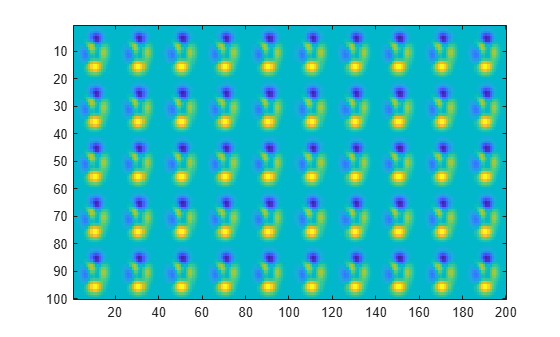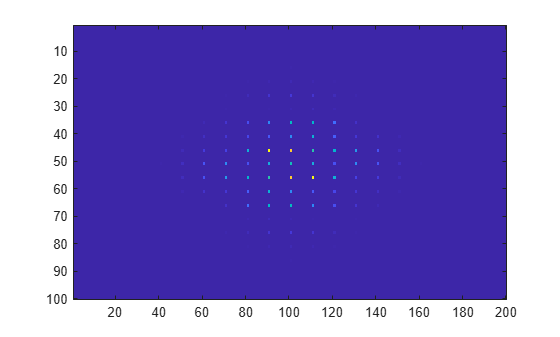# fft2

## 语法

``Y = fft2(X)``
``Y = fft2(X,m,n)``

## 说明

``Y = fft2(X)` 使用快速傅里叶变换算法返回矩阵的二维傅里叶变换，这等同于计算 `fft(fft(X).').'`。如果 `X` 是一个多维数组，`fft2` 将采用高于 2 的每个维度的二维变换。输出 `Y` 的大小与 `X` 相同。`

``Y = fft2(X,m,n)` 将截断 `X` 或用尾随零填充 `X`，以便在计算变换之前形成 `m`×`n` 矩阵。`Y` 是 `m`×`n` 矩阵。如果 `X` 是一个多维数组，`fft2` 将根据 `m` 和 `n` 决定 `X` 的前两个维度的形状。`

## 示例

```P = peaks(20); X = repmat(P,[5 10]); imagesc(X)``````Y = fft2(X); imagesc(abs(fftshift(Y)))``````Y = fft2(X,2^nextpow2(100),2^nextpow2(200)); imagesc(abs(fftshift(Y)));```## 详细信息

### 二维傅里叶变换

`${Y}_{p+1,q+1}=\sum _{j=0}^{m-1}\sum _{k=0}^{n-1}{\omega }_{m}^{jp}{\omega }_{n}^{kq}{X}_{j+1,k+1}$`

ωm 和 ωn 是复单位根：

`$\begin{array}{l}{\omega }_{m}={e}^{-2\pi i/m}\\ {\omega }_{n}={e}^{-2\pi i/n}\end{array}$`

i 是虚数单位。p 和 j 是值范围从 0 到 m–1 的索引，q 和 k 是值范围从 0 到 n–1 的索引。此公式将 X 和 Y 的索引平移 1 位，以反映 MATLAB® 中的矩阵索引。